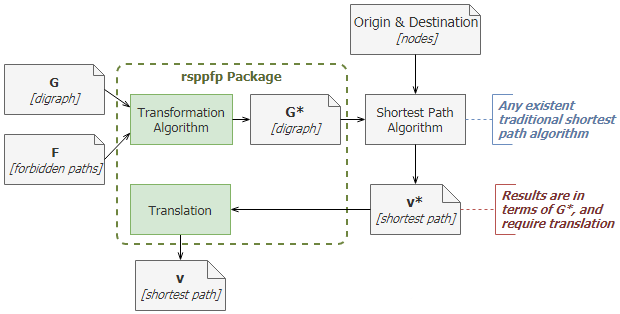The rsppfp package implements different algorithms for transforming graphs in the Shortest Path Problem with Forbidden Paths (SPPFP). This problem is an important concept in the field of graph theories, and it is a variant of the traditional shortest path problem. In here, there is an additional constrait that takes the form of a finite set of forbidden paths (arc sequences of at least three nodes) that cannot be part of any solution path.

This problem is solved by transforming the original graph G and its set of forbidden paths F, generating a new graph G* in which traditional shortest path algorithms can be applied, and obtain solutions that abide to the restrictions. This approach has a number of advantages:

• It allows solving the original shortest path problem in G* with algorithms that efficiently manage time and processing resources constraints, having been implemented in a plethora of languages.
• The resulting G* is highly compatible with existing libraries, and can be used as input data for other, more complex problems and researches.
• In many cases -i.e. logistics- G and F remain unchanged for long periods of time. Thus, the transformation is completed only once, and G* can be stored along with the original graph. A new conversion is required only on the rare cases where the graph, or its forbidden paths, are modified.
• The input data is provided as common data frames, increasing the versatility of this package.

This solving process is illustrated in Figure 1, using a paper notation to indicate input and output data. Even more, rsppfp scope and key functionalities are also highlighted.Figure 1. Flow diagram showcasing an SPPFP solving process, and highlighting rsppfp package’s contribution.

## Algorithms

rsppfp implements two different algorithms, each one suited for different situations:

1. Villeneuve and Desaulniers (2005) proposed the first algorithm. In this case, the set F must be known beforehand. This transformation is slightly fast, but generates bigger graphs G*. Each forbidden path can be of different size, but no sub-path (of at least three nodes long) can be part of another forbidden path.
2. Hsu et al. ‘Backward Construction’ (2009) solves the restriction of sub-paths in the forbidden paths, and generates smaller graphs G*, by adding less new nodes and arcs. However, this algorithm is slightly slower.

Both algorithms are analyzed using 27 graphs, randomly generated. The complete benchmark evaluation can be found here.

## Installation

As from 2018-11-22 you can install rsppfp directly from CRAN, using:

install.packages("rsppfp")

You can install the development version of rsppfp from GitHub with:

# install.packages("devtools")
devtools::install_github("melvidoni/rsppfp")